# Catch up on the latest AI articles# Using Adversarial Attacks To Improve Accuracy!

3 main points
✔️ Improve the accuracy of the model by adding adversarial perturbations in a loss reducing manner
✔️ Improve the accuracy of the model by adding noise to the object for regions, called patches
✔️ Propose a Robust Pattern to explain the model by removing the norm constraint on the noise.

written by Camilo Pestana, Wei Liu, David Glance, Robyn Owens, Ajmal Mian
(Submitted on June 2021)
Subjects: Computer Vision and Pattern Recognition (cs.CV)

The images used in this article are from the paper, the introductory slides, or were created based on them.

## Outline of Research

In this research, we used the idea of an adversarial attack to improve the accuracy of the model. The perturbation to improve the accuracy of the model is called Assistive Signals, and it is added to a part of the image to be identified to improve the accuracy. The Assistive Signals are also considered to indicate the bias of the ML model towards a particular pattern of a real object (Robust Pattern).

## Research Background

### What is an adversarial attack?

The adversarial attack is a general term for a method of processing the input so that the machine learning model produces incorrect output for a given input. It has been shown that there is the noise that can cause a machine learning model to produce incorrect outputs simply by putting small noise on the input data that is not visible to the human eye.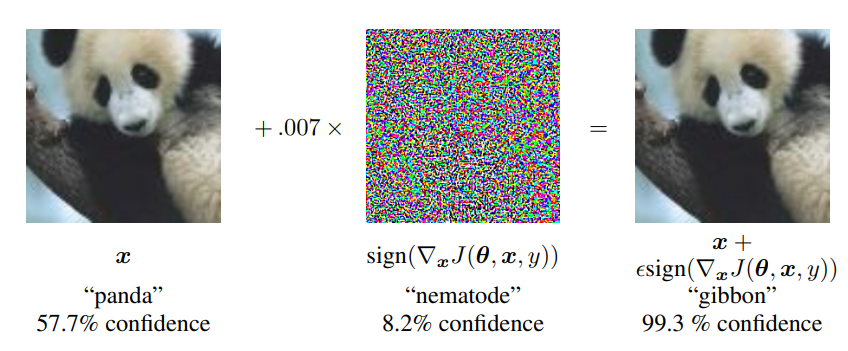The above figure is a famous illustration of an adversarial attack. By adding noise to the input panda image, we transform it to the image on the right. The human eye cannot tell the difference between the images, but the machine learning model correctly identifies the first image as a panda, but the transformed image is identified as a gibbon. Thus, adversarial attacks can mislead the output of the model.

So how does an adversarial attack seek this kind of noise? Most of the adversarial attacks are performed by adding noise such that the loss of correctly classified images becomes large. Specifically, the attacker solves the following optimization problem.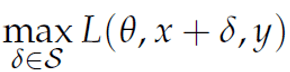where $\delta$ is the noise to be sought, $x$ is the input data, $y$ is the correct label corresponding to the input data, and $L$ is the loss function. Usually, the loss function is computed by the parameters of the model, the input data, and the correct label, so the attacker modifies the input data so that the loss is large when it is classified to the correct label.

## What are Assistive Signals?

So what is Assistive Signals, which uses the idea of adversarial attacks to improve the accuracy of a model? The answer is simple: adversarial attacks add noise that increases the loss when the model correctly classifies, but Assistive Signals adds noise that decreases the loss when the model correctly classifies. In other words, the noise is added in such a way that the model becomes easier to classify. In other words, Assistive Signals adds noise that makes it easier for the model to classify. This is probably why it is called Assistive Signals.

### use case

Assistive Signals require that the correct class of the input data is known before classification, to apply noise to the input data so that it is correctly classified. Therefore, it is not possible to prepare unknown data. So, in what situations can we use Assistive Signals?

The authors envision a scene in which one of the use cases is the identification of objects in physical space. Specifically, the task is to identify cars running in the city. In this kind of task, it is easy to identify the car by attaching a kind of sticker that represents noise to the car itself.

Thus, it is not practical to add noise to the entire input image, as we want to use it in the task of correctly classifying real-world objects. Therefore, the authors propose to add noise only to small regions, called patches. In this way, the noise can be reproduced with something like a sticker, as described above.

### Algorithm of signal generation

Specifically, Assistive Signals are generated by the following procedure.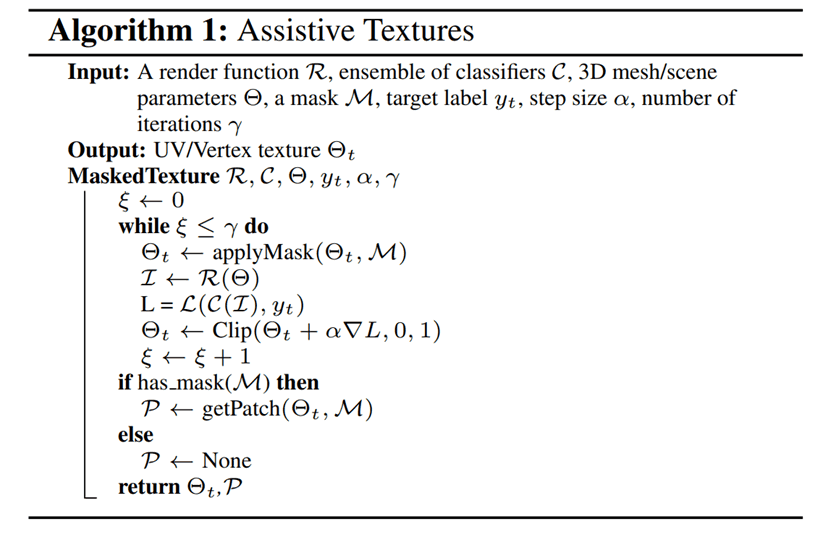The authors consider physical space as a target, but in this case, the experiment is performed on a 3D space that simulates it. Therefore, there is a parameter $\Theta$ in the simulation. This parameter determines the illumination, the viewing angle, and so on.

The above procedure is an operation to find the noise for an image of an object in 3D space taken from a certain camera. After the image is captured, noise is added in the direction of decreasing loss for a predetermined number of times. At this time, to avoid adding noise to the whole image, the operation "applyMask" is performed before adding noise. By this operation, noise is added to only a part of the image.

## experiment

From here, we will examine the effectiveness of Assistive Signals. First, we will compare the results when Assistive Signals are added and when they are not.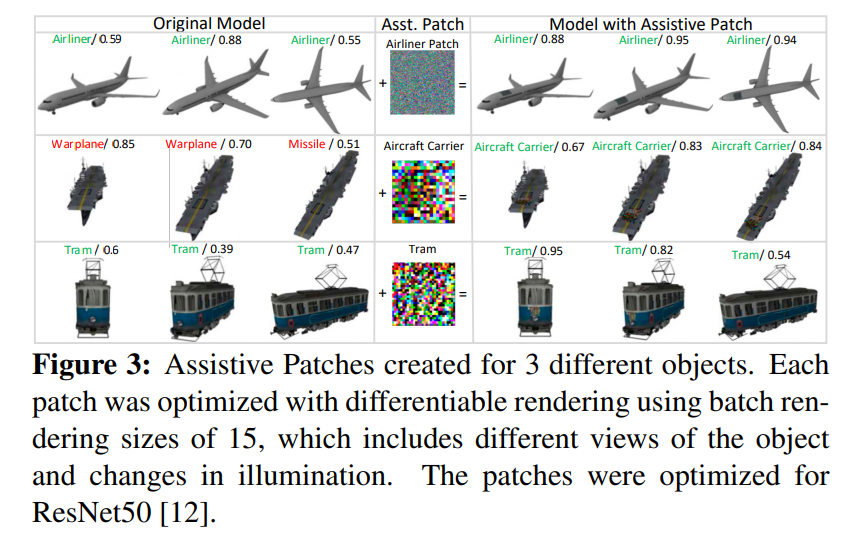As shown in the figure above, when Assistive Signals (made into a patch is called Assistive Patch) are added, you can see that the accuracy is improved.

Next, we examine the difference between adding noise to the entire image and adding noise to a portion of the image.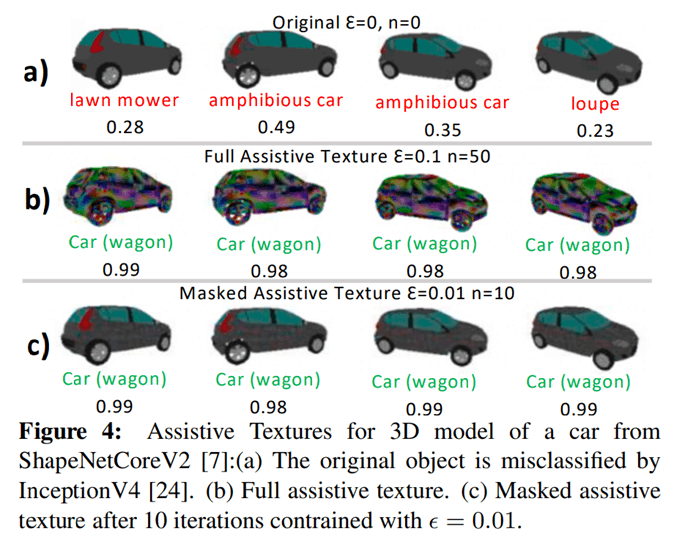As shown in the above figure, there is almost no difference in accuracy between the case where noise is added to the entire image (b) and the case where noise is added to a part of the image (c). Therefore, when adding noise to improve the accuracy, it is enough to add noise to a part of the image.

Finally, I will explain the concept of Robust Patterns. Normally, when we put noise, we put noise that is so small that it cannot be seen by humans, but we examined what kind of results Assistive Signals would produce if we removed the restriction on the size of the noise. We also consider putting noise over the whole image instead of just patches.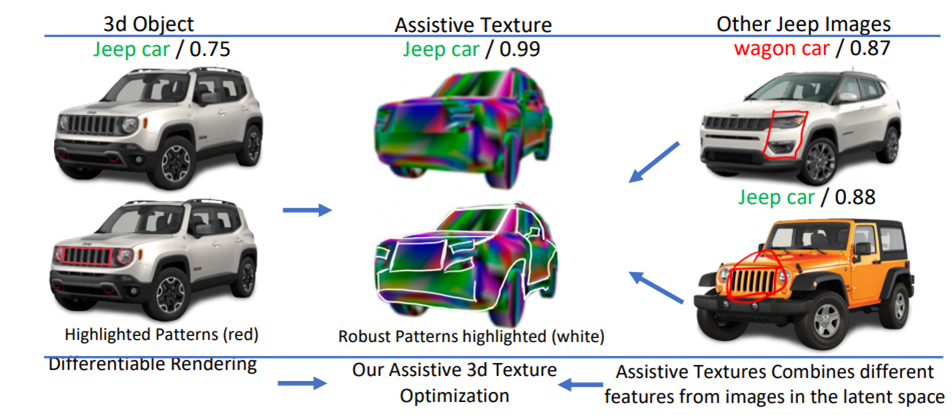The result is shown in the figure above. This result shows that the model learns the square lights and large grille from the other data, rather than the round lights and elongated grille from the current input data, as features that identify the car as a Jeep car. The authors call this result the Robust Pattern and believe it may provide useful information for model explanatory properties.

## summary

I introduced something called Assistive Signals, which uses the idea of adversarial attacks to improve the accuracy of the model. I found it to be a very interesting concept, and I'd like to see some experimental results in real physical space.

If you have any suggestions for improvement of the content of the article,